The Volume of a Cuboctahedron (II)

A cuboctahedron is an Archimedean solid. It is generated by truncating the vertices of a cube or of an octahedron at 1/2 edge-length.A cuboctahedron is an Archimedean solid. It can be seen as made by cutting off the corners of a cube.

The cube and the octahedron are dual polyhedra. There are 6 square faces on the cuboctahedron, one for each vertex of the octahedron. There are 8 equilateral triangular faces, one for each face of the octahedron.

We are going to calculate the volume of an octahedron of edge-length 1 starting from the volume of an octahedron.The volume of an octahedron is four times the volume of a tetrahedron. It is easy to calculate and then we can get the volume of a tetrahedron.

If a cuboctahedron has edge-length 1, the octahedron that contains it is:The volume of an octahedron of edge-length 2 is:

To calculate the volume of a cuboctahedron we have to subtract from the volume of the octahedron the volume of the 6 pyramids that we cut off.

Each pyramid is half octahedron. The volume of one of these pyramids is: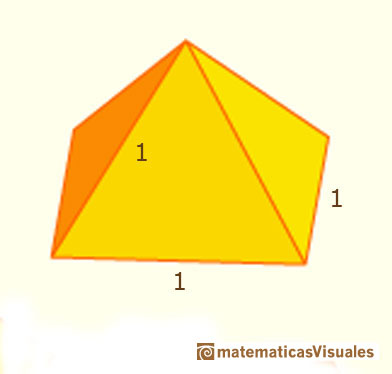Now, we can calculate the volume of the cuboctahedron (what we subtract, 6 pyramids, may be reasssembled into three octahedron of edge-length 1)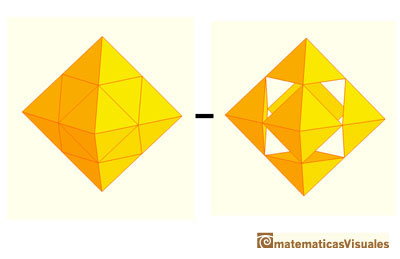Then the volume of a cuboctahedron of edge-length a is:

Origami cuboctahedron made with six business cards (Instructions in Origami Resource Center, click over the image to get the instructions)

Cuboctahedron and octahedron made with rubber bands and paper: (Go to Resources: Polyhedra made with rubber bands and paper if you want to build polyhedra using this technique.)It was Durer the first to publish plane nets of polyhedra. In his book 'Underweysung der Messung' ('Four Books of Measurement', published in 1525) the author draw plane developments of several Platonic and Archimedean solids, for example, this cuboctahedron:REFERENCES

Hugo Steinhaus - Mathematical Snapshots - Oxford University Press - Third Edition (p. 197)
Magnus Wenninger - 'Polyhedron Models', Cambridge University Press.
Peter R. Cromwell - 'Polyhedra', Cambridge University Press, 1999.
H.Martin Cundy and A.P. Rollet, 'Mathematical Models', Oxford University Press, Second Edition, 1961 (p. 87).
W.W. Rouse Ball and H.S.M. Coxeter - 'Matematical Recreations & Essays', The MacMillan Company, 1947.
Luca Pacioli - De divina proportione - (La divina proporción) Ediciones Akal, 4ª edición, 2004. Spanish translation by Juan Calatrava.The volume of an octahedron is four times the volume of a tetrahedron. It is easy to calculate and then we can get the volume of a tetrahedron.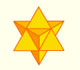The stellated octahedron was drawn by Leonardo for Luca Pacioli's book 'De Divina Proportione'. A hundred years later, Kepler named it stella octangula.The volume of a tetrahedron is one third of the prism that contains it.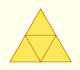The first drawing of a plane net of a regular tetrahedron was published by Dürer in his book 'Underweysung der Messung' ('Four Books of Measurement'), published in 1525 .We study different prisms and we can see how they develop into a plane net. Then we explain how to calculate the lateral surface area.Plane nets of prisms with a regular base with different side number cut by an oblique plane.We study different cylinders and we can see how they develop into a plane. Then we explain how to calculate the lateral surface area.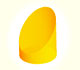We study different cylinders cut by an oblique plane. The section that we get is an ellipse.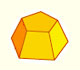Plane net of pyramids and pyramidal frustrum. How to calculate the lateral surface area.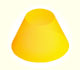Plane developments of cones and conical frustum. How to calculate the lateral surface area.Plane developments of cones cut by an oblique plane. The section is an ellipse.The first drawing of a plane net of a regular dodecahedron was published by Dürer in his book 'Underweysung der Messung' ('Four Books of Measurement'), published in 1525 .The twelve vertices of an icosahedron lie in three golden rectangles. Then we can calculate the volume of an icosahedronSome properties of this platonic solid and how it is related to the golden ratio. Constructing dodecahedra using different techniques.The truncated octahedron is an Archimedean solid. It has 8 regular hexagonal faces and 6 square faces. Its volume can be calculated knowing the volume of an octahedron.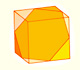We can cut in half a cube by a plane and get a section that is a regular hexagon. Using eight of this pieces we can made a truncated octahedron.Using eight half cubes we can make a truncated octahedron. The cube tesselate the space an so do the truncated octahedron. We can calculate the volume of a truncated octahedron.These polyhedra pack together to fill space, forming a 3 dimensional space tessellation or tilling.The truncated octahedron is an Archimedean solid. It has 8 regular hexagonal faces and 6 square faces. Its volume can be calculated knowing the volume of an octahedron.Leonardo da Vinci made several drawings of polyhedra for Luca Pacioli's book 'De divina proportione'. Here we can see an adaptation of the truncated octahedron.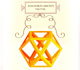Leonardo da Vinci made several drawings of polyhedra for Luca Pacioli's book 'De divina proportione'. Here we can see an adaptation of the cuboctahedron.Leonardo da Vinci made several drawings of polyhedra for Luca Pacioli's book 'De divina proportione'. Here we can see an adaptation of the dodecahedron.Leonardo da Vinci made several drawings of polyhedra for Luca Pacioli's book 'De divina proportione'. Here we can see an adaptation of the stellated octahedron (stella octangula).The truncated tetrahedron is an Archimedean solid made by 4 triangles and 4 hexagons.When you truncate a cube you get a truncated cube and a cuboctahedron. If you truncate an octahedron you get a truncated octahedron and a cuboctahedron.You can chamfer a cube and then you get a polyhedron similar (but not equal) to a truncated octahedron. You can get also a rhombic dodecahedron.